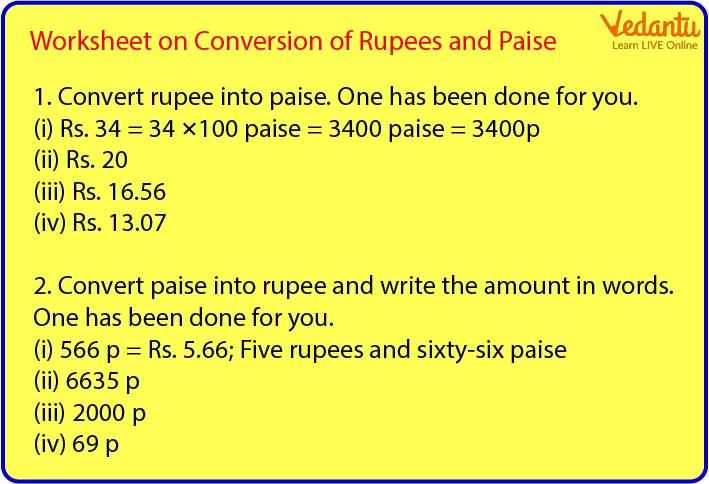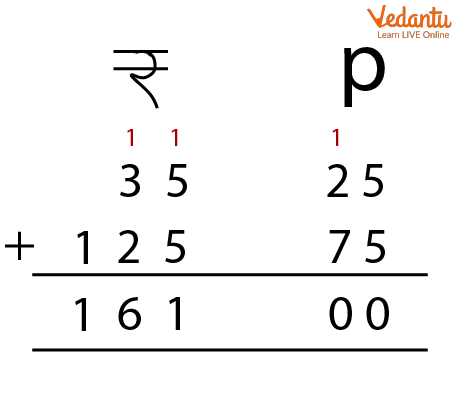Courses
Courses for Kids
Free study material
Free LIVE classes
More

# Convert Rupees into PaiseLIVE
Join Vedantu’s FREE Mastercalss

## Introduction

Every country has its own currency. The currency of India is Indian Rupee. We all see and deal with Rupees in our daily lives. Whenever we go shopping, watch a movie, play games, buy gifts or whatnot, we need money. We see different coins and notes of different denominations.Coin Denomination

A rupee has a hundred paise in it. We also write 1 Rupee= 100 Paise or 100 Paise= 1 Rupee. Let us understand the concept of rupees and paise. We will also understand the concept of converting rupees into paise.

## How to Convert Rupees into Paise?

One Rupee= 100 Paise or we can also write it like 100 Paise= 1 Rupee. The rupee and paise are separated by a dot (.). Paise is written to the right of the dot and rupee is written to the left of the dot. In ancient times, paise had great value but now only rupees are in existence. 10 Rupee is written as Rs. 10.00 and 10 Rupee and 75 Paise is written as Rs. 10.75.

The conversion of Rupees into Paise happens when we multiply the rupees by a hundred. Let us take a few examples to understand this better.

Convert Rs. 4 into Paise= 4 is to be multiplied by 100= 400 Paise.

Convert Rs. 9 into Paise= 9 is to be multiplied by 100= 900 Paise.

Convert Rs. 12 into Paise= 12 is to be multiplied by 100= 1200 Paise

## How to Convert Paise into Rupees?

As we understood the concept of converting rupees into paise, let us now understand how we convert paise into rupees.

While converting paise into rupees we divide paise by 100. Converting paise into rupee formula is to divide the paise by hundred. . Let us take a few examples to understand this better.

Convert 200 paise into rupees = \$\dfrac{200}{100}\$ = Rs. 2

Convert 500 paise into rupees = \$\dfrac{500}{100}\$ = Rs. 5

Convert 800 paise into rupees = \$\dfrac{800}{100}\$ = Rs. 8

## How to Convert Paise into Rupees Using Decimal?

We can also convert paise into rupees using decimals. Let us see a few examples to understand this better.

Convert 5 paise into rupees using decimals = \$\dfrac{5}{100}\$ = Rs. 0.05

Convert 75 paise into rupees using decimals = \$\dfrac{75}{100}\$ = Rs. 0.75

Convert 20 paise into rupees using decimals = \$\dfrac{20}{100}\$ = Rs. 0.20As we know that we write rupee and paise by separating them with a dot. In the example of adding 35.25 and 125.75, firstly we write them in vertical addition form. We add the paise first and then the rupees. The final answer to this question would be 161.00 Rupees.

The same pattern is followed in the subtraction as well. Firstly we arrange them vertically and then subtract the small amount from the large amount.

## Solved Examples

Q1. Convert 42 Rupee into Paise?

Ans: To convert rupee into paise, we have to multiply rupee with 100 paise. In this question, we have to multiply 42 by a hundred. 42 Rupee multiplied by 100 would be 4200 Paise.

Q2. Convert 700 paise into rupee?

Ans: To convert paise into rupee, we have to divide paise by 100. In this question, we have to divide 700 paise by 100. 700/100= 7 Rupee.

Q1. How to convert 7 Rupees into Paise?

Ans: 700 Paise

Q2. How to convert 800 Paise into Rupees?

Ans: 8 Rupee

## Summary

We deal with rupees and paise in our daily lives. Nothing can be bought without money. Money is required to do everything. In ancient times, paise was also of use but now only rupees are in use. The formulas to convert rupee into paisa and paise into rupee are to be understood well. These concepts are combined with multiplication and division as well. Children must know how to deal with money. These concepts have to be taught well to the kids.

Last updated date: 19th Sep 2023
Total views: 121.5k
Views today: 1.21k

## FAQs on Convert Rupees into Paise

1. How to convert the rupee into paise? Give an example to understand it better.

To convert a rupee into a paisa we must know that one rupee is equal to hundred paise. A rupee has to be multiplied by a hundred in order to get an equal paisa to the value. Let us look at a few examples.

To convert rs 5 into Paisa we have to multiply 5 by 100. 5×100= 500 paise.

Let us take another example of 30 Rs.

Multiply 30 by 100 to get the value of paisa. 30×100= 3000 paise.

2. What is converting paise into rupees calculator? Give an example to explain it better.

Convert paise into rupee calculator is used to convert paise into rupees. To convert a passion into a rupee we have to divide the value of the paisa by 100. Let us take your examples to understand this better.

To convert 300 paise into rupees we divide \$\dfrac{300}{100}\$ which equals 00.03 Rs.

To convert 600 paise into rupees we divide \$\dfrac{600}{100}\$ which is equal to Rs. 00.06.

To convert 800 paise into rupees we divide \$\dfrac{800}{100}\$ which is equal to Rs. 00.08.